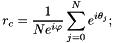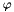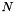pyclustering.nnet.sync.sync_network Class Reference

Model of oscillatory network that is based on the Kuramoto model of synchronization. More...Inheritance diagram for pyclustering.nnet.sync.sync_network:Collaboration diagram for pyclustering.nnet.sync.sync_network:

## Public Member Functions

def __init__ (self, num_osc, weight=1, frequency=0, type_conn=conn_type.ALL_TO_ALL, representation=conn_represent.MATRIX, initial_phases=initial_type.RANDOM_GAUSSIAN, ccore=True)
Constructor of oscillatory network is based on Kuramoto model. More...

def __del__ (self)
Destructor of oscillatory network is based on Kuramoto model.

def sync_order (self)
Calculates current level of global synchorization (order parameter) in the network. More...

def sync_local_order (self)
Calculates current level of local (partial) synchronization in the network. More...

def simulate (self, steps, time, solution=solve_type.FAST, collect_dynamic=True)
Performs static simulation of Sync oscillatory network. More...

def simulate_dynamic (self, order=0.998, solution=solve_type.FAST, collect_dynamic=False, step=0.1, int_step=0.01, threshold_changes=0.0000001)
Performs dynamic simulation of the network until stop condition is not reached. More...

def simulate_static (self, steps, time, solution=solve_type.FAST, collect_dynamic=False)
Performs static simulation of oscillatory network. More...

def get_neighbors (self, index)
Finds neighbors of the oscillator with specified index. More...

def has_connection (self, i, j)
Returns True if there is connection between i and j oscillators and False - if connection doesn't exist. More...Public Member Functions inherited from pyclustering.nnet.network
def height (self)
Height of the network grid (that is defined by amout of oscillators in each column), this value is zero in case of non-grid structure. More...

def width (self)
Width of the network grid, this value is zero in case of non-grid structure. More...

def structure (self)
Type of network structure that is used for connecting oscillators.

def __init__ (self, num_osc, type_conn=conn_type.ALL_TO_ALL, conn_repr=conn_represent.MATRIX, height=None, width=None)
Constructor of the network. More...

def __len__ (self)
Returns size of the network that is defined by amount of oscillators.

def has_connection (self, i, j)
Returns True if there is connection between i and j oscillators and False - if connection doesn't exist. More...

def set_connection (self, i, j)
Couples two specified oscillators in the network with dynamic connections. More...

def get_neighbors (self, index)
Finds neighbors of the oscillator with specified index. More...

## Detailed Description

Model of oscillatory network that is based on the Kuramoto model of synchronization.

CCORE option can be used to use the pyclustering core - C/C++ shared library for processing that significantly increases performance.

Definition at line 722 of file sync.py.

## ◆ __init__()

 def pyclustering.nnet.sync.sync_network.__init__ ( self, num_osc, weight = 1, frequency = 0, type_conn = conn_type.ALL_TO_ALL, representation = conn_represent.MATRIX, initial_phases = initial_type.RANDOM_GAUSSIAN, ccore = True )

Constructor of oscillatory network is based on Kuramoto model.

Parameters
 [in] num_osc (uint): Number of oscillators in the network. [in] weight (double): Coupling strength of the links between oscillators. [in] frequency (double): Multiplier of internal frequency of the oscillators. [in] type_conn (conn_type): Type of connection between oscillators in the network (all-to-all, grid, bidirectional list, etc.). [in] representation (conn_represent): Internal representation of connection in the network: matrix or list. [in] initial_phases (initial_type): Type of initialization of initial phases of oscillators (random, uniformly distributed, etc.). [in] ccore (bool): If True simulation is performed by CCORE library (C++ implementation of pyclustering).

Definition at line 730 of file sync.py.

## ◆ get_neighbors()

 def pyclustering.nnet.sync.sync_network.get_neighbors ( self, index )

Finds neighbors of the oscillator with specified index.

Parameters
 [in] index (uint): index of oscillator for which neighbors should be found in the network.
Returns
(list) Indexes of neighbors of the specified oscillator.

Definition at line 1036 of file sync.py.

## ◆ has_connection()

 def pyclustering.nnet.sync.sync_network.has_connection ( self, i, j )

Returns True if there is connection between i and j oscillators and False - if connection doesn't exist.

Parameters
 [in] i (uint): index of an oscillator in the network. [in] j (uint): index of an oscillator in the network.

Definition at line 1052 of file sync.py.

## ◆ simulate()

 def pyclustering.nnet.sync.sync_network.simulate ( self, steps, time, solution = solve_type.FAST, collect_dynamic = True )

Performs static simulation of Sync oscillatory network.

Parameters
 [in] steps (uint): Number steps of simulations during simulation. [in] time (double): Time of simulation. [in] solution (solve_type): Type of solution (solving). [in] collect_dynamic (bool): If True - returns whole dynamic of oscillatory network, otherwise returns only last values of dynamics.
Returns
(list) Dynamic of oscillatory network. If argument 'collect_dynamic' = True, than return dynamic for the whole simulation time, otherwise returns only last values (last step of simulation) of dynamic.
simulate_dynamic()
simulate_static()

Definition at line 851 of file sync.py.

## ◆ simulate_dynamic()

 def pyclustering.nnet.sync.sync_network.simulate_dynamic ( self, order = 0.998, solution = solve_type.FAST, collect_dynamic = False, step = 0.1, int_step = 0.01, threshold_changes = 0.0000001 )

Performs dynamic simulation of the network until stop condition is not reached.

Stop condition is defined by input argument 'order'.

Parameters
 [in] order (double): Order of process synchronization, distributed 0..1. [in] solution (solve_type): Type of solution. [in] collect_dynamic (bool): If True - returns whole dynamic of oscillatory network, otherwise returns only last values of dynamics. [in] step (double): Time step of one iteration of simulation. [in] int_step (double): Integration step, should be less than step. [in] threshold_changes (double): Additional stop condition that helps prevent infinite simulation, defines limit of changes of oscillators between current and previous steps.
Returns
(list) Dynamic of oscillatory network. If argument 'collect_dynamic' = True, than return dynamic for the whole simulation time, otherwise returns only last values (last step of simulation) of dynamic.
simulate()
simulate_static()

Definition at line 871 of file sync.py.

## ◆ simulate_static()

 def pyclustering.nnet.sync.sync_network.simulate_static ( self, steps, time, solution = solve_type.FAST, collect_dynamic = False )

Performs static simulation of oscillatory network.

Parameters
 [in] steps (uint): Number steps of simulations during simulation. [in] time (double): Time of simulation. [in] solution (solve_type): Type of solution. [in] collect_dynamic (bool): If True - returns whole dynamic of oscillatory network, otherwise returns only last values of dynamics.
Returns
(list) Dynamic of oscillatory network. If argument 'collect_dynamic' = True, than return dynamic for the whole simulation time, otherwise returns only last values (last step of simulation) of dynamic.
simulate()
simulate_dynamic()

Definition at line 938 of file sync.py.

## ◆ sync_local_order()

 def pyclustering.nnet.sync.sync_network.sync_local_order ( self )

Calculates current level of local (partial) synchronization in the network.

Returns
(double) Level of local (partial) synchronization.
sync_order()

Definition at line 814 of file sync.py.

Referenced by pyclustering.nnet.sync.sync_network.simulate_dynamic().

## ◆ sync_order()

 def pyclustering.nnet.sync.sync_network.sync_order ( self )

Calculates current level of global synchorization (order parameter) in the network.

This parameter is tend 1.0 when the oscillatory network close to global synchronization and it tend to 0.0 when desynchronization is observed in the network. Order parameter is calculated using following equation:whereis a average phase coordinate in the network,is an amount of oscillators in the network.

Example:

oscillatory_network = sync(16, type_conn = conn_type.ALL_TO_ALL);
output_dynamic = oscillatory_network.simulate_static(100, 10);
if (oscillatory_network.sync_order() < 0.9): print("Global synchronization is not reached yet.");
else: print("Global synchronization is reached.");
Returns
(double) Level of global synchronization (order parameter).